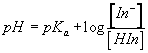HOME | INTRODUCTION | PRELAB | PROCEDURE | REPORT | PRINTABLE PDFSpectrophotometric Determination of pH : Introduction

The pH of an unknown solution can be determined by addition of an acid/base indicator of known Ka and spectrophotometric measurement of the relative concentrations of the acid and base forms of the indicator. This method has been used as a basis for continuous shipboard monitoring of seawater pH.
The relationship between the two forms of the indicator in an aqueous solution is described by the equilibrium

HIn + H2O ↔ H3O+ + In-

for whichandThus if pKa is known and [In-]/[HIn] is measured, the pH of the solution can be calculated.

In this exercise the indicator is bromocresol green, with Ka = 1.6 x 10-5. The spectra of the acid and base forms of bromocresol green overlap. The absorbance of the solution at a given wavelength is equal to the sum of the absorbances of the individual components in a mixture. For two overlapping components the absorbance must be measured at two wavelengths.

A1 = ea1bCacid + eb1bCbase
A2 = ea2bCacid + eb2bCbase

where the subscripts 1 and 2 indicate the two wavelengths, b is the path length of the spectrophotometer and Cacid and Cbase are the concentrations of the acid and base forms of the indicator.

To determine the amount of each form in a mixture from the measured absorbances requires knowledge of the molar absorptivity of each form. In a preliminary experiment the absorption spectra of the acid and base forms of the indicator are measured separately. The wavelength of maximum absorbance for each component is identified, and the molar absorptivities of each component at these two wavelengths are determined. The absorbance of the solution of unknown pH is measured at the same two wavelengths, and the concentrations of the two forms of the indicator in this solution are calculated by solving the two simultaneous equations describing the solution absorbances at the two wavelengths.